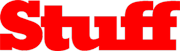Notice: Undefined index: HTTP_REFERER in /var/www/html/n-gage.com/livesite/wp-content/plugins/geographical-redirect/geo-redirect.php on line 37
One messaging app that fits all! | n-gage messenger

# One messaging app that fits all!Check your smartphone right now and chances are that there’ll be no less than three messaging apps on it.

That includes the humble sms application which is pretty much the blueprint from where WhatsApp and others of its ilk sprouted from. With a burgeoning set of options that never ceases to stop, you could say we are a generation spoilt for choices. With options like Snapchat, Hike, Facebook messenger among others popping up continuously, we’ve also ingeniously formed circles of friends based on the app that we use to communicate with them. Boom! how’s that for an epiphany? But, staying true to the topic at hand, the guys at N-gage.com thought up of an app that loosley put, is a universal remote of sorts, and more. Claimed to be the world’s first 360 degree lifestyle chat-app, N-gage allows you to send texts, videos, doodles, audio recordings, emoticons (vanilla and customisable ones with audio in them!) and a lot more across other messaging app such as the ones mentioned above. But, that is just the tip of the iceberg. This is one app that can do more, a lot more.

The biggest trick up N-gage’s sleeve is most definitely its ability to accommodate almost all the popular messaging apps in play. But basically, this iOS, Android and even PC compatible app is essentially a messaging app. But it offers enough features to put a smile on Ethan Hunt. Mission, impossible? Au contraire. Using the app you can send password-protected content (texts, video, audio, yada yada… keep up!), put a timer on it in a “this message will self-destruct in 10 seconds” type of homage, and even use an option that lets you scramble text so that peeping Toms remain none-the-wiser. You can also choose a variety of themes to make the UI suit your style, which includes modifying various icons and what not. Got a mate in Germany and you no ‘Sprechen Sie Deutsch’? No problem, as an in-house translation feature will make sure the conversation flows like Bavarian beer. There’s even a ‘Lifestyle’ section that lets you connect with fellow topic-lovers if you get our drift. With more and more features being constantly added, this just might well be on it’s way to be the only app you’ll need for staying in touch, virtually.

Read the original post on the Stuff website: https://www.stuff.tv/in/news/one-messaging-app-fits-all

1 year ago

1

1 year ago

1

1 year ago

1

1 year ago

1

1 year ago

1

1 year ago

1

1 year ago

1

1 year ago

1

1 year ago

1

1 year ago

)

1 year ago

1 year ago

1

1 year ago

pTFiqsmp’; waitfor delay ‘0:0:13’ —

1 year ago

../../../../../../../../../../../../../../../proc/version

1 year ago

WEB-INF/web.xml

1 year ago

../../../../../../../../../../windows/win.ini

1 year ago

unexisting/../../../../../../../../../../windows/win.ini.\.\.\.\.\.\.\.\.\.\.\.\.\.\.\.\.\.\.\.\.\.\.\.\.\.\.\.\.\.\.\.\.\.\.\.\.\.\.\.\.\.\.\.\.\.\.\.\.\.\.\.\.\.\.\.\.\.\.\.\.\.\.\.\.\.\.\.\.\.\.\.\.\.\.\.\.\.\.\.\.\.\.\.\.\.\.\.\.\.\.\.\.\.\.\.\.\.\.\.\.\.\.\.\.\.\.\.\.\.\.\.\.\.\.\.\.\.\.\.\.\.\.\.\.\.\.\.\.\.\.\.\.\.\.\.\.\.\.\.\.\.\.\.\.\.\.\.\.\.\.\.\.\.\.\.\.\.\.\.\.\.\.\.\.\.\.\.\.\.\.\.\.\.\.\.\.\.\.\.\.\.\.\.\.\.\.\.\.\.\.\.\.\.\.\.\.\.\.\.\.\.\.\.\.\.\.\.\.\.\.\.\.\.\.\.\.\.\.\.\.\.\.\.\.\.\.\.\.\.\.\.\.\.\.\.\.\.\.\.\.\.\.\.\.\.\.\.\.\.\.\.\.\.\.\.\.\.\.\.\.\.\.\.\.\.\.\.\.\.\.\.\.\.\.\.\.\.\.\.\.\.\.\.\.\.\.\.\.\.\.\.\.\.\.\.\.\.\.\.\.\.\.\.\.\.\.\.\.\.\.\.\.\.\.\.\.\.\.\.\.\.\.\.\.\.\.\.\.\.\.\.\.\.\.\.\.\.\.\.\.\.\.\.\.\.\.\.\.\.\.\.\.\.\.\.\.\.\.\.\.\.\.\.\.\.\.\.\.\.\.\.\.\.\.\.\.\.\.\.\.\.\.\.\.\.\.\.\.\.\.\.\.\.\.\.\.\.\.\.\.\.\.\.\.\.\.\.\.\.\.\.\.\.\.\.\.\.\.\.\.\.\.\.\.\.\.\.\.\.\.\.\.\.\.\.\.\.\.\.\.\.\.\.\.\.\.\.\.\.\.\.\.\.\.\.\.\.\.\.\.\.\.\.\.\.\.\.\.\.\.\.\.\.\.\.\.\.\.\.\.\.\.\.\.\.\.\.\.\.\.\.\.\.\.\.\.\.\.\.\.\.\.\.\.\.\.\.\.\.\.\.\.\.\.\.\.\.\.\.\.\.\.\.\.\.\.\.\.\.\.\.\.\.\.\.\.\.\.\.\.\.\.\.\.\.\.\.\.\.\.\.\.\.\.\.\.\.\.\.\.\.\.\.\.\.\.\.\.\.\.\.\.\.\.\.\.\.\.\.\.\.\.\.\.\.\.\.\.\.\.\.\.\.\.\.\.\.\.\.\.\.\.\.\.\.\.\.\.\.\.\.\.\.\.\.\.\.\.\.\.\.\.\.\.\.\.\.\.\.\.\.\.\.\.\.\.\.\.\.\.\.\.\.\.\.\.\.\.\.\.\.\.\.\.\.\.\.\.\.\.\.\.\.\.\.\.\.\.\.\.\.\.\.\.\.\.\.\.\.\.\.\.\.\.\.\.\.\.\.\.\.\.\.\.\.\.\.\.\.\.\.\.\.\.\.\.\.\.\.\.\.\.\.\.\.\.\.\.\.\.\.\.\.\.\.\.\.\.\.\.\.\.\.\.\.\.\.\.\.\.\.\.\.\.\.\.\.\.\.\.\.\.\.\.\.\.\.\.\.\.\.\.\.\.\.\.\.\.\.\.\.\.\.\.\.\.\.\.\.\.\.\.\.\.\.\.\.\.\.\.\.\.\.\.\.\.\.\.\.\.\.\.\.\.\.\.\.\.\.\.\.\.\.\.\.\.\.\.\.\.\.\.\.\.\.\.\.\.\.\.\.\.\.\.\.\.\.\.\.\.\.\.\.\.\.\.\.\.\.\.\.\.\.\.\.\.\.\.\.\.\.\.\.\.\.\.\.\.\.\.\.\.\.\.\.\.\.\.\.\.\.\.\.\.\.\.\.\.\.\.\.\.\.\.\.\.\.\.\.\.\.\.\.\.\.\.\.\.\.\.\.\.\.\.\.\.\.\.\.\.\.\.\.\.\.\.\.\.\.\.\.\.\.\.\.\.\.\.\.\.\.\.\.\.\.\.\.\.\.\.\.\.\.\.\.\.\.\.\.\.\.\.\.\.\.\.\.\.\.\.\.\.\.\.\.\.\.\.\.\.\.\.\.\.\.\.\.\.\.\.\.\.\.\.\.\.\.\.\.\.\.\.\.\.\.\.\.\.\.\.\.\.\.\.\.\.\.\.\.\.\.\.\.\.\.\.\.\.\.\.\.\.\.\.\.\.\.\.\.\.\.\.\.\.\.\.\.\.\.\.\.\.\.\.\.\.\.\.\.\.\.\.\.\.\.\.\.\.\.\.\.\.\.\.\.\.\.\.\.\.\.\.\.\.\.\.\.\.\.\.\.\.\.\.\.\.\.\.\.\.\.\.\.\.\.\.\.\.\.\.\.\.\.\.\.\.\.\.\.\.\.\.\.\.\.\.\.\.\.\.\.\.\.\.\.\.\.\.\.\.\.\.\.\.\.\.\.\.\.\.\.\.\.\.\.\.\.\.\.\.\.\.\.\.\.\.\.\.\.\.\.\.\.\.\.\.\.\.\.\.\.\.\.\.\.\.\.\.\.\.\.\.\.\.\.\.\.\.\.\.\.\.\.\.\.\.\.\.\.\.\.\.\.\.\.\.\.\.\.\.\.\.\.\.\.\.\.\.\.\.\.\.\.\.\.\.\.\.\.\.\.\.\.\.\.\.\.\.\.\.\.\.\.\.\.\.\.\.\.\.\.\.\.\.\.\.\.\.\.\.\.\.\.\.\.\.\.\.\.\.\.\.\.\.\.\.\.\.\.\.\.\.\.\.\.\.\.\.\.\.\.\.\.\.\.\.\.\.\.\.\.\.\.\.\.\.\.\.\.\.\.\.\.\.\.\.\.\.\.\.\.\.\.\.\.\.\.\.\.\.\.\.\.\.\.\.\.\.\.\.\.\.\.\.\.\.\.\.\.\.\.\.\.\.\.\.\.\.\.\.\.\.\.\.\.\.\.\.\.\.\.\.\.\.\.\.\.\.\.\.\.\.\.\.\.\.\.\.\.\.\.\.\.\.\.\.\.\.\.\.\.\.\.\.\.\.\.\.\.\.\.\.\.\.\.\.\.\.\.\.\.\.\.\.\.\.\.\.\.\.\.\.\.\.\.\.\.\.\.\.\.\.\.\.\.\.\.\.\.\.\.\.\.\.\.\.\.\.\.\.\.\.\.\.\.\.\.\.\.\.\.\.\.\.\.\.\.\.\.\.\.\.\.\.\.\.\.\.\.\.\.\.\.\.\.\.\.\.\.\.\.\.\.\.\.\.\.\.\.\.\.\.\.\.\.\.\.\.\.\.\.\.\.\.\.\.\.\.\.\.\.\.\.\.\.\.\.\.\.\.\.\.\.\.\.\.\.\.\.\.\.\.\.\.\.\.\.\.\.\.\.\.\.\.\.\.\.\.\.\.\.\.\.\.\.\.\.\.\.\.\.\.\.\.\.\.\.\.\.\.\.\.\.\.\.\.\.\.\.\.\.\.\.\.\.\.\.\.\.\.\.\.\.\.\.\.\.\.\.\.\.\.\.\.\.\.\.\.\.\.\.\.\.\.\.\.\.\.\.\.\.\.\.\.\.\.\.\.\.\.\.\.\.\.\.\.\.\.\.\.\.\.\.\.\.\.\.\.\.\.\.\.\.\.\.\.\.\.\.\.\.\.\.\.\.\.\.\.\.\.\.\.\.\.\.\.\.\.\.\.\.\.\.\.\.\.\.\.\.\.\.\.\.\.\.\.\.\.\.\.\.\.\.\.\.\.\.\.\.\.\.\.\.\.\.\.\.\.\.\.\.\.\.\.\.\.\.\.\.\.\.\.\.\.\.\.\.\.\.\.\.\.\.\.\.\.\.\.\.\.\.\.\.\.\.\.\.\.\.\.\.\.\.\.\.\.\.\.\.\.\.\.\.\.\.\.\.\.\.\.\.\.\.\.\.\.\.\.\.\.\.\.\.\.\.\.\.\.\.\.\.\.\.\.\.\.\.\.\.\.\.\.\.\.\.\.\.\.\.\.\.\.\.\.\.\.\.\.\.\.\.\.\.\.\.\.\.\.\.\.\.\.\.\.\.\.\.\.\.\.\.\.\.\.\.\.\.\.\.\.\.\.\.\.\.\.\.\.\.\.\.\.\.\.\.\.\.\.\.\.\.\.\.\.\.\.\.\.\.\.\.\.\.\.\.\.\.\.\.\.\.\.\.\.\.\.\.\.\.\.\.\.\.\.\.\.\.\.\.\.\.\.\.\.\.\.\.\.\.\.\.\.\.\.\.\.\.\.\.\.\.\.\.\.\.\.\.\.\.\.\.\.\.\.\.\.\.\.\.\.\.\.\.\.\.\.\.\.\.\.\.\.\.\.\.\.\.\.\.\.\.\.\.\.\.\.\.\.\.\

1 year ago

1

9 months ago

Hi, very nice website, cheers!
——————————————————
Need cheap and reliable hosting? Our shared plans start at \$10 for an year and VPS plans for \$6/Mo.
——————————————————
Check here: https://www.good-webhosting.com/